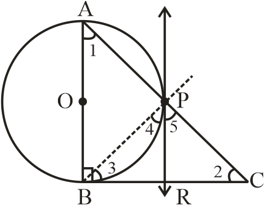#### In a right triangle ABC in which B = , a circle is drawn with AB as diameter intersecting the hypotenuse AC and P. Prove that the tangent to the circle at P bisects BC.SolutionLet O be the center of the given circle.
Suppose P meet BC at point R
Construction: Join point P and B
To Prove: BR = RC
Proof:   [Given]
In ABC
[  Sum of interior angle of a triangle is 180°]

Also              [  tangent and chord made equal angles in alternate segment]

[  angle in semi circle formed is 90°]

Equal equation (i) and (ii) we get

[Side opposite to equal angles are equal]
Also,    PR = BR         …..(iv)   [  tangents drawn to a circle from external point are equal]

From equation (iii) and (iv)
BR = RC

Hence Proved